Courses

# Vapour Pressure of Liquid Solutions Class 12 Notes | EduRev

## JEE : Vapour Pressure of Liquid Solutions Class 12 Notes | EduRev

The document Vapour Pressure of Liquid Solutions Class 12 Notes | EduRev is a part of the JEE Course Chemistry Class 12.
All you need of JEE at this link: JEE

CONCEPT OF VAPOUR PRESSURE
When a pure liquid is kept in closed vessel (in which no air is present) the liquid evaporates to give the vapours.
The molecules of the liquid which possess high kinetic energy have a tendency to change to vapour state.
After sometime a dynamic equilibrium is established between liquid and vapours.

At Equilibrium
rate of evaporation = rate of condensation.
H2O (ℓ) ⇌ H2O (g)
The pressure that the vapours exert at equilibrium on the walls of the container or on the surface of the liquid is called the vapour pressure of the liquid at that temperature, Figure (a).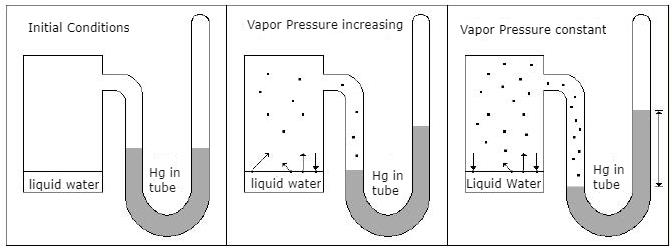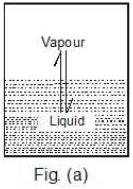Case 1: What would happen to the vapour pressure of the liquid if we take a bigger container, Figure (b) or a wider container, Figure (c).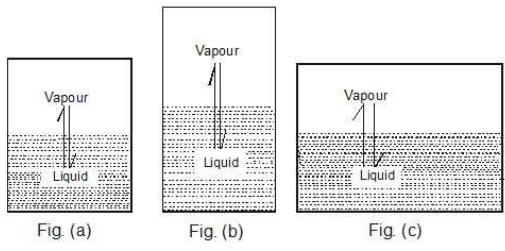Key concept: When the liquid is in equilibrium with vapours,
Liquid ⇌ Vapours,
the Kp of the system is Kp = Pv,
where Pv is the vapour pressure.
We know that Kp for a given chemical equilibrium is a constant and only depends on temperature. Therefore the vapour pressure of the liquid is a constant and does not depend on the nature of the vessel used and that it only depends on temperature.

Case 2: Take a vessel with the same liquid as in case 1 and cover it with a glass plate (of negligible weight) having a hole in it. Would the vapour pressure be the same now?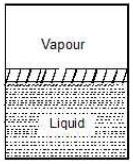Key concept: Well, the liquid system is the same and the temperature is also same. Therefore, the vapour pressure should be same. The vapour pressure of a liquid is independent of the surface area exposed on top of the liquid surface provided the surface area exposed should not be zero.

The vapour pressure of liquid depends upon

• Nature of the liquid
• Temperature

(i) Nature of the Liquid: When the intermolecular forces of attractions are stronger then the vapour pressure will be low because less number of molecules can leave the liquid.
Out of C2H5OH, CH3OCH3, CH3CHO the one with highest vapour pressure is CH3OCH3 and the one with lowest vapour pressure is C2H5OH because in C2H5OH there is hydrogen bonding leading to strongest intermolecular forces where as in CH3OCH3 there is Vander Waals forces leading to weakest intermolecular forces.
Decreasing order of vapour pressure of few liquids is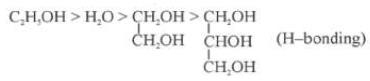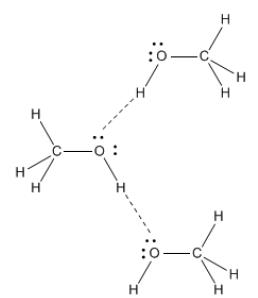Fig: H-Bonding in Methanol

(ii) Temperature: Higher the temperature, greater would be the vapour pressure.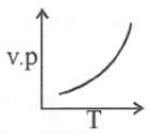This is because when temperature is raised, kinetic energy of the molecules increase and therefore the number of molecules leaving surface of liquid, is large.
The Clausius Clapeyron equation tells very clearly that as temperature increases the right hand side remains highly and thereby vapour pressure increases.
This equation is,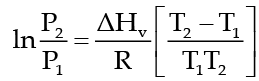which tells as to how vapour pressure changes with nature of liquid and temperature when vapour pressure is low then the temperature at which the liquid would boil will be high. In the above equation P1 and P2 are vapour pressure of liquid at T1 and T2 respectively, ΔHv, heat of vaporization and R is gas constant.

VAPOUR PRESSURE OF LIQUID SOLUTIONS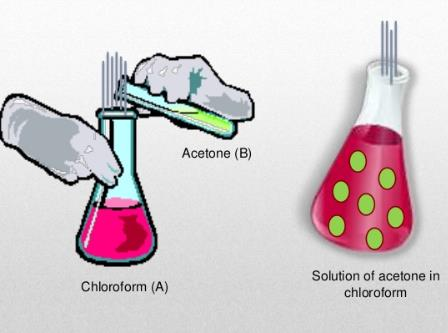Fig: Example of Liquid in Liquid Solution

Liquid Solution is formed when we dissolve a solid, liquid or gas in a particular liquid solvent.
Vapour Pressure of liquid solutions is defined as the pressure exerted by the vapours on the liquid solvent when kept in equilibrium and a certain temperature.

• It varies with the nature of liquid and temperature of the surroundings.

Some characteristics of the vapour pressure of liquid solutions are as follows:

• The pure liquid has more vapour pressure as compared to liquid’s solution.
Example: take two beaker one filled with water and other with lemon juice and water, you’ll find that the beaker filled with water has more vapour pressure
• Vapour pressure is inversely proportional to forces of attraction between molecules of a liquid
• Vapour pressure increases with increase in temperature, as molecules gain kinetic energy and vapourise briskly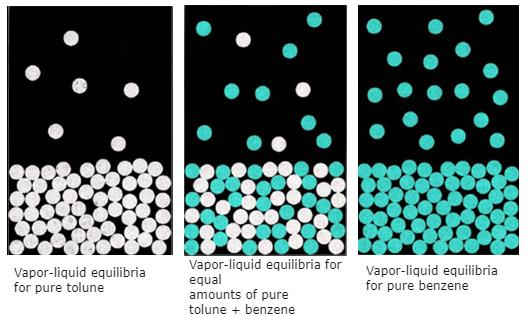Fig: Vapour pressure equilibrium in different solution

Liquid solution can contain volatile solute and solvent as well. In most cases, the solvent is volatile in nature and the solute left out to be non-volatile.
Vapour pressure can be evaluated in two cases which are:

• Vapour pressure of liquid-liquid solutions that is, solute and solvent both are in liquid phase
• Vapour pressure of solid- liquid solutions that is, solute is in solid state and solvent is in liquid phase

Vapour Pressure of Liquid-Liquid Solutions
To find out vapour pressure of the liquid-liquid solution, we take two volatile liquid solutions and name their liquid component as A and B. When we place the volatile liquid with their components in a closed vessel, we find that equilibrium is established between the liquid phase and vapour phase.
Suppose Ptotal is the overall vapour pressure at an equilibrium state and let PA and PB be the partial vapour pressures of components A and B respectively.
Applying Dalton’s law of partial pressure the total vapour pressure of the solution will be
Psolution = PA + PB
Where PA and PB are the partial vapour pressures of A and B.
Adding further, the mole fraction of respective components is xA and xrespectively.
To evaluate vapour pressure of volatile liquids, we use Raoult’s Law.

Offer running on EduRev: Apply code STAYHOME200 to get INR 200 off on our premium plan EduRev Infinity!

## Chemistry Class 12

121 videos|250 docs|199 tests

,

,

,

,

,

,

,

,

,

,

,

,

,

,

,

,

,

,

,

,

,

;